# ACCRINT Function

Calculates the accrued interest for a security that pays interest on a periodic basis

## What is the ACCRINT Function?

The ACCRINT Function is an Excel Financial function. The function will calculate the accrued interest for a security that pays interest on a periodic basis.

ACCRINT helps users calculate the accrued interest on a security, such as a bond, when that security is sold or is transferred to a new owner on a date other than the issue date or on a date that is an interest payment date.

The ACCRINT function was introduced in MS Excel 2007 and hence is not available in earlier versions.

### Formula

=ACCRINT(issue, first_interest, settlement, rate, par, frequency, [basis], [calc_method])

The ACCRINT function uses the following arguments:

1. Issue (required argument) – This is the security’s issue date.
2. First_interest (required argument) – This is the first interest date of the security.
3. Settlement (required argument) – The security’s settlement date. It is the date after the issue date when the security is traded to the buyer.
4. Rate (required argument) – The security’s annual coupon rate.
5. Par (required argument) – The security’s par value. If omitted by the user, the function will take the par value as \$1,000.
6. Frequency (required argument) – This is the number of coupons payments per year. The function will take for annual payments, frequency = 1; for semiannual, frequency = 2; for quarterly, frequency = 4.
7. Basis (optional argument) – This is the kind of day count that is used for calculating the interest on a given security. If we omit the argument, the basis is set to 0. Basis can be any of the following values:

BasisDay Count basis
0 or omittedUS(NASD) 30/360
1Actual/actual
2Actual/360
3Actual/365
4European 30/360

8. Calc_method (optional argument) – It is either 0 (calculates the accrued interest from first_interest_date to settlement_date) or 1 (calculates the accrued interest from issue_date to settlement_date).

### How to use the ACCRINT Function in Excel?

To understand the uses of the ACCRINT function, let’s consider a few examples:

#### Example 1

Suppose we are given the following details:

• Issue date: 2017/01/01
• First interest date: 2017/03/31
• Settlement date: 2022/02/15
• Rate: 6.25%
• Par: 10,000
• Frequency: 4
• Basis – 2: 2
• Calculation method: 1

The formula to use is: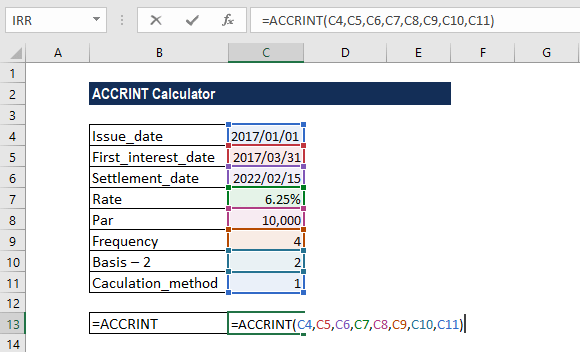The result we got is \$3,203.13, which is the interest on maturity that we will receive.

The function calculated ACCRINT using the formula below: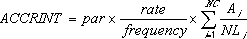Where:

• Ai is the number of accrued days for the quasi-coupon period within the odd period.
• NC is the number of quasi-coupon periods that fit in the odd period. Fraction is raised to the next whole number.
• NLi is the normal length in days of the quasi-coupon period within the odd period.

We get the result below: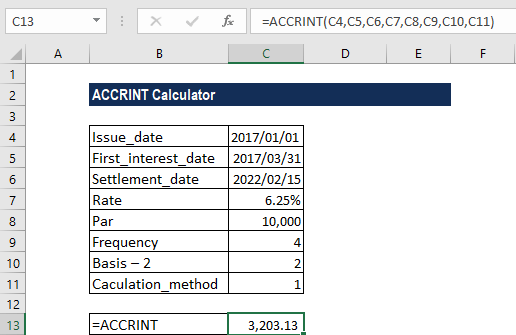#### Example 2 – Using DATE with ACCRINT

If the dates given are not in date format, we can use the DATE function along with ACCRINT to get the interest receivable on maturity. Suppose we are given the following data: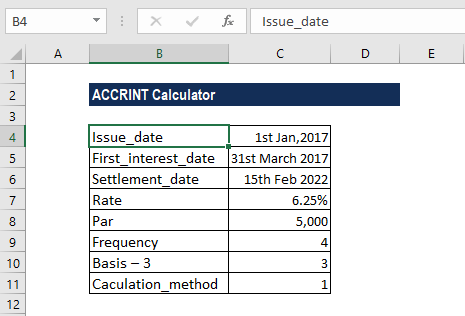The formula used was: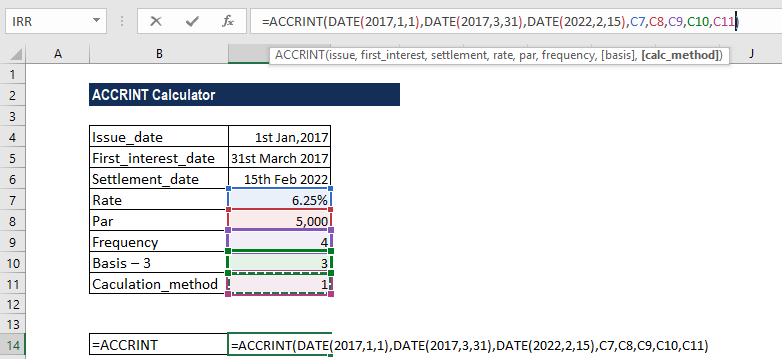We get the result below: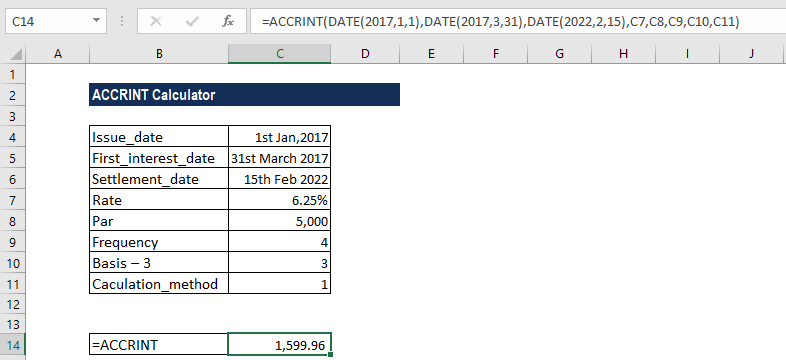### Things to remember about the ACCRINT Function

1. #NUM! error – Occurs when:
• The given rate argument is ≤ 0 or the provided [par] argument is ≤ 0.
• The given frequency argument is not equal to 1, 2, or 4.
• We provided issue ≥ settlement.
• The given basis argument is not equal to 0, 1, 2, 3, or 4.
1. #VALUE! error – Occurs when:
• The given issue, first_interest, or settlement arguments are not valid dates.
• Any of the arguments provided is non-numeric.
1. When we input the issue and settlement dates, they should be entered as either:
• References to cells that contain dates; or
• Dates that are returned from formulas; or
• If we attempt to input these date arguments as text, Excel may incorrectly interpret them, due to different date systems or date interpretation settings.

Thanks for reading CFI’s guide to important Excel functions! By taking the time to learn and master these functions, you’ll significantly speed up your financial analysis. To learn more, check out these additional CFI resources:

• Excel Functions for Finance